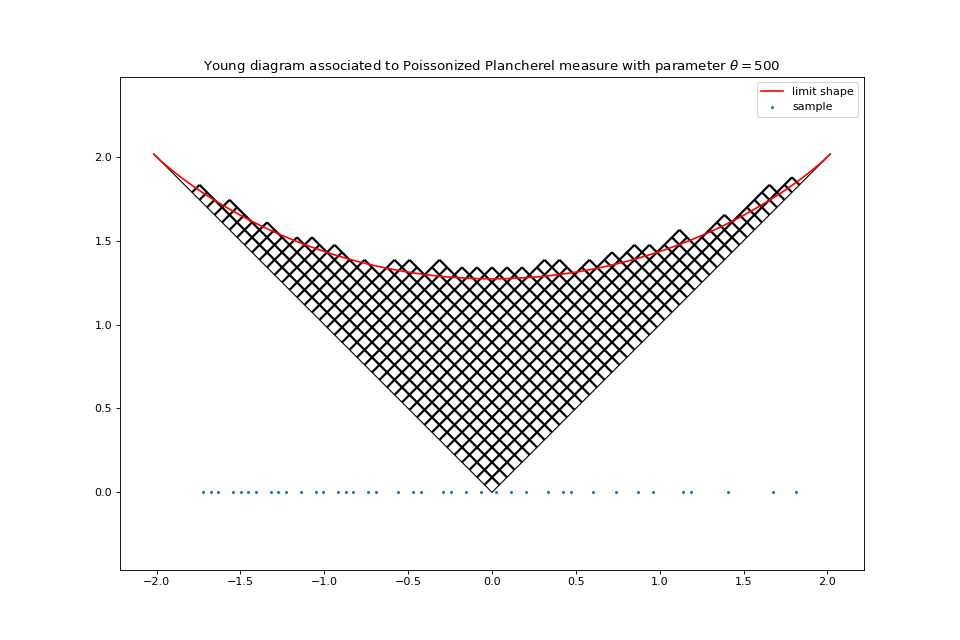# Poissonized Plancherel measure¶

The poissonized Plancherel measure is a measure on partitions $$\lambda=(\lambda_1 \geq \lambda_2 \geq \cdots \geq 0)\in \mathbb{N}^{\mathbb{N}^*}$$. Samples from this measure can be obtained in the following way

• Sample $$N \sim \mathcal{P}(\theta)$$

• Sample a uniform permutation $$\sigma\in \mathfrak{S}_N$$

• Compute the sorting tableau $$P$$ associated to the RSK (Robinson-Schensted-Knuth correspondence) applied to $$\sigma$$

• Consider only the shape $$\lambda$$ of $$P$$.

Finally, the point process formed by $$\{\lambda_i - i + \frac12\}_{i\geq 1}$$ is a DPP on $$\mathbb{Z}+\frac12$$.

from dppy.exotic_dpps import PoissonizedPlancherel

theta = 500  # Poisson parameter
pp = PoissonizedPlancherel(theta=theta)
pp.sample()
pp.plot_diagram(normalization=True)Fig. 53 Poissonized Plancherel measure